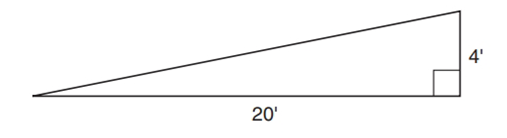Chapter 4.3, Problem 41EElementary Geometry For College St...

7th Edition
Alexander + 2 others
ISBN: 9781337614085

Solutions

Chapter
SectionElementary Geometry For College St...

7th Edition
Alexander + 2 others
ISBN: 9781337614085
Textbook Problem

In Exercises 40 to 41, you will need to use the square root ( ) function of your calculator.A walk-up ramp runs 20 ft horizontally while rising 4 ft. Use a calculator to approximate its length to the nearest tenth of a foot.To determine

To find:

The length of the walk-up ramp.

Explanation

Calculation:

Given:

Let the walk-up ramp denoted by ABC.

By using Pythagorean Theorem

AC2=AB2+BC2=20

Still sussing out bartleby?

Check out a sample textbook solution.

See a sample solution

The Solution to Your Study Problems

Bartleby provides explanations to thousands of textbook problems written by our experts, many with advanced degrees!

Get Started

Find y and y. y=xlnx

Calculus: Early Transcendentals

Fill in the blanks. 4. An equation of the circle with center C(a, b) and radius r is given by __________.

Applied Calculus for the Managerial, Life, and Social Sciences: A Brief Approach

Convert the expressions in Exercises 6584 to power form. 23x1.2x2.13

Finite Mathematics and Applied Calculus (MindTap Course List)

Find all solutions of the equation. 21. x2 + 2x + 5 = 0

Single Variable Calculus: Early Transcendentals

For a given M 0, the corresponding in the definition of limx012x4= is: a) 2M4 b) 2M4 c) 12M4 d) 12M4

Study Guide for Stewart's Single Variable Calculus: Early Transcendentals, 8th

Which is the best graph of r = 1 − sin θ for 0 ≤ θ ≤ π?

Study Guide for Stewart's Multivariable Calculus, 8th